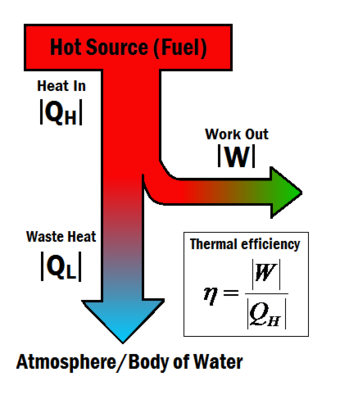# Thermal efficiencyFigure 1: The amount of work output for a given amount of heat gives a system its thermal efficiency.

Heat engines turn heat into work. The thermal efficiency expresses the fraction of heat that becomes useful work. The thermal efficiency is represented by the symbol , and can be calculated using the equation:

Where:

is the useful work and

is the total heat energy input from the hot source.

Heat engines often operate at around 30% to 50% efficiency, due to practical limitations. It is impossible for heat engines to achieve 100% thermal efficiency () according to the Second law of thermodynamics. This is impossible because some waste heat is always produced produced in a heat engine, shown in Figure 1 by the term. Although complete efficiency in a heat engine is impossible, there are many ways to increase a system's overall efficiency.

### An Example

If 200 joules of thermal energy as heat is input (), and the engine does 80 J of work (), then the efficiency is 80J/200J, which is 40% efficient.

This same result can be gained by measuring the waste heat of the engine. For example, if 200 J is put into the engine, and observe 120 J of waste heat, then 80 J of work must have been done, giving 40% efficiency.

## Carnot Efficiency

main article

There is a maximum attainable efficiency of a heat engine which was derived by physicist Sadi Carnot. Following laws of thermodynamics the equation for this turns out to be

Where

is the temperature of the cold 'sink' and

is the temperature of the heat reservoir.

This describes the efficiency of an idealized engine, which in reality is impossible to achieve. From this equation, the lower the sink temperature or the higher the source temperature , the more work is available from the heat engine. The energy for work comes from a decrease in the total energy of the fluid used in the system. Therefore the greater the temperature change, the greater this decrease in the fluid and thus the greater energy available to do work is.